# Red diplomas

The numbers of students with honors in 2013 and 2014 are in ratio 40:49. How big is the year-on-year percentage increase?

p =  22.5 %

### Step-by-step explanation:Did you find an error or inaccuracy? Feel free to write us. Thank you!Tips to related online calculators
Need help to calculate sum, simplify or multiply fractions? Try our fraction calculator.
Check out our ratio calculator.

## Related math problems and questions:

• Fixed expenses 2013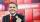Since 2013, the Slovak government plans to tax more small one person businesses. Instead of fixed expences 40% will be fixed expences 40% of gross income up to 420 Eur. Calculate what percentage of fixed expences will in 2013 pays from gross income 1569 EAfter two hours and 40 minutes, the job is finished. Compared to last year, we were 40 minutes faster. What is the percentage increase to our performance?
• Soup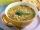47% of pupils at the school to eat in the canteen, 1/47 of students who eat in the canteen eat lunch soup. a) What percentage of pupils who eat in the canteen and eat soup for lunch? b) What percentage of all pupils of school eat lunch soup?
• FortyForty five of the 80 students were girls. What is the ratio of girls to boys?
• Tulips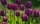Of the 120 tulips in the garden, 45 are red, 66 are yellow, the rest are brindle. What percentage of tulips in the garden are brindle?
• Boys and girlsIf the ratio of male & female teachers is 2/5 & it is in proportion of the male & female students, how many are girls if there are 42 boys?
• There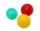There are two numbers on the screen - one in the blue and the other in the red box. In the beginning, both numbers are the same. With each beep, both numbers increase - by 1 in the blue field and by 3 in the red field. At one point, the number 49 appears
• Wheat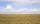The rectangle shaped field with dimensions 1268 m and 745 m harvested last year was 4959 q wheat. (1 q = 1 quintal = 100 kg). During the year, it was necessary to fix the pipe and therefore did kick wide 4 m parallel to the side of the field 745 m, where
• The ratio 4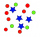The ratio of two number is 5:4 if 40% of the first number is 12, what will be 50% of the second number?
• Three-fifthsThree-fifths of the school's students are girls. What percentage are boys?
• What percent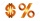What percent of 51 3/5 is 47 2/5?
• The farmer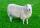The farmer had 140 sheep. For the next year, she decided to change the number of sheep in ratio 10: 7. How many sheep will he have then?
• Percentage increaseIncrease number 400 by 3.5%
• Report 2A School reports students to teacher ratio of 6:1. If there are 45 teachers in the School, how many students are there?
• PC disksPeter has 45 disks in three colors. One-fifth of the disks are blue, red are twice more than the white. How much is blue, red and white disks?
• The rawThe raw data presented here are the scores (out of 100 marks) of a market survey regarding the acceptability of new product launched by a company for random sample of 50 respondents: 40 45 41 45 45 30 30 8 48 25 26 9 23 24 26 29 8 40 41 42 39 35 18 25 35
• Oranges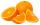Mother divided her three children's oranges in a ratio of 6:5:4. Two children gave 45 oranges. How many oranges were there?